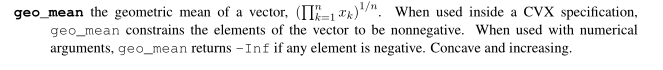# (x*y)^(1/2) Disciplined convex programming error: Invalid quadratic form(s): not a square

(x*y)^(1/2) Disciplined convex programming error:
Invalid quadratic form(s): not a square.

variables x y
(x*y)^(1/2) Disciplined convex programming error:
Invalid quadratic form(s): not a square.

Use geo_mean([x y]).